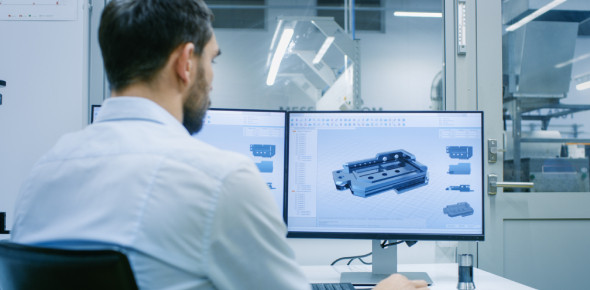# Quiz: Introduction To Engineering Design Questions

50 Questions | Total Attempts: 757Settings.

Related Topics
• 1.
Which of the following disciplines involves the complex analysis, design, and development of solutions to technical problems?
• A.

Mathematics

• B.

Engineering

• C.

Science

• D.

Medicine

• 2.
A designer will often keep _________________ of his or her best work to show off to prospective clients.
• A.

A VHS tape

• B.

A portfolio

• C.

A design brief

• D.

An engineer's notebook

• 3.
The shape pictured below is called ________________
• A.

A circle

• B.

An ellipse

• C.

A spline

• D.

A parallelogram

• 4.
The hand-written messages on the fire truck sketch below are examples of ____________.
• A.

Annotations

• B.

Tolerances

• C.

Thumbnails

• D.

Design briefs

• 5.
__________ is a type of drawing that is done freehand and without the use of drawing tools.
• A.

Computer-Aided Design

• B.

Sketching

• C.

Prototyping

• D.

Isometric

• 6.
The image below is referred to as a ____________________.
• A.

Pie chart

• B.

Line chart

• C.

Column chart

• D.

Bar graph

• 7.
What type of chart is pictured below?
• A.

Column Chart

• B.

Pie Chart

• C.

Line Graph

• D.

Bar Graph

• 8.
What type of full-scale physical model would an engineer use to demonstrate how a design functions?
• A.

Prototype

• B.

Inventor Assembly

• C.

Conceptual Model

• D.

Appearance Model

• 9.
A full-size clay model of a new car body design would be considered a _______________.
• A.

Prototype

• B.

Mock-up

• C.

Conceptual model

• D.

Mathematical model

• 10.
What 3D solid modeling feature would be used to establish a two-dimentional drawing surface that is tangent to the outside of a sphere?
• A.

Sketch Plane

• B.

Revolve

• C.

Origin

• D.

Work Plane

• 11.
The one (1) inch diameter THRU hole dimension shown on the drawing below represents the ______________ dimension.
• A.

A datum

• B.

A size

• C.

A location

• D.

An aligned

• 12.
Study the image. The section lines in regions A and C in the front view of the drawing indicate __________________.
• A.

The cutting plane contacts material only in regions A and C.

• B.

The cutting plane contacts material only in region B.

• C.

That regions A and C are hollow.

• D.

That regions A and C are finished surfaces.

• 13.
_____________ often take the form of dimensions, symbols, or notes that are added to a drawing to provide details and give clarity.
• A.

Auxiliaries

• B.

Annotations

• C.

Schematics

• D.

Sections

• 14.
Which figure below contains a multiview drawing that is associated with the isometric pictorial in the center?
• A.

Figure 1

• B.

Figure 2

• C.

Figure 3

• D.

Figure 4

• 15.
When a professional delivers a formal presentation, he or she should _______________.
• A.

Dress informally.

• B.

Read the contents of each slide to the audience

• C.

Maintain eye contact with the audience.

• D.

Speak in a monotone voce.

• 16.
Graphs, charts, mock-ups, prototypes, and computer graphics represent what type of presentation aid?
• A.

PowerPoint

• B.

• C.

Written Documentation

• D.

Visual

• 17.
What kind of line is 1.1?
• A.

Extension Line

• B.

Object Line

• C.

Hidden Line

• D.

Center Line

• 18.
What kind of line is 1.2?
• A.

Extension Line

• B.

Dimension Line

• C.

Object Line

• D.

Section Line

• 19.
What kind of line is 1.3?
• A.

Extension Line

• B.

Object Line

• C.

Hidden Line

• D.

Center Line

• 20.
What kind of line is 1.4?
• A.

Extension Line

• B.

Object Line

• C.

Hidden Line

• D.

Center Line

• 21.
What kind of line is 1.5?
• A.

• B.

Section Line

• C.

Dimension Line

• D.

Construction Line

• 22.
What kind of line is 1.6?
• A.

Object Line

• B.

Center Line

• C.

Hidden Line

• D.

Construction Line

• 23.
What is the height from the base of the part to the center of the .250 diameter hole?
• 24.
What is the depth of the .250 diameter hole?
• 25.
What is the overall width of the part?